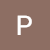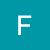# Formulas For Speed Time And Distance## Formulas of Speed, Time and Distance:-

Speed measures of how quickly an object moves from one place to another. Speed is equal to the distance traveled divided by the time taken.

By using speed, time and distance formulas we can easily find the three values

## Formulas of Speed, Time and Distance

Distance = Speed x Time

 Speed =DistanceTime
 Time =DistanceSpeedFormula for Conversion of Km/hr to m/sec where x is in Km/hr

1 km = 1000m

1 h = 3600 s

So 1km /h = $\frac{1000}{3600} \$

= $\frac{5}{18} \$

 Y m/sec =X x 5m/sec 18

Formula for Conversion of m/sec to Km/hr where x is in m/sec

1 m =$\frac{1}{1000} \$ km;

1 sec = $\frac{1}{3600} \$ hr

1m/sec = $\frac{3600}{1000} \$

= $\frac{18}{5} \$

 Y km/hr =X x 18km/hr 5

## Equal Distance at two speeds

An object covers equal distance  at speed S1 and other equal distance at speed S2 then his average speed for the distance is (2S1S2)/(S1 + S2)### Train based Formulas of Speed, Time and Distance-

• S = Speed of Train
• S = Speed of Object
• L = Length of Train
• L = Length of Object#### Case 1 –

When Train Crosses a Stationary Object with no Length(e.g. Pole) in time t

• ST = $\frac{L_T}{t} \$#### Case 2 –

When Train Crosses a Stationary Object with Length L(e.g. Train Platform) in time t

• ST = (L+LO) / t#### Case 3 –

When Train Crosses a Moving Object with no Length (e.g. Car has negligible length) in time t

• Objects moving in Opposite directions
• (S+ SO) = LT/ t
• Objects moving in Same directions
• (S– SO) = LT/ t#### Case 3 –

When Train Crosses a Moving Object with no Length (e.g. Car has negligible length) in time t

• Objects moving in Opposite directions
• (S+ SO) = LT/ t
• Objects moving in Same directions
• (S– SO) = LT/ t#### Case 4 –

When Train Crosses a Moving Object with Length LO (e.g. Another Train treated as an object) in time t

• Objects(Trains) moving in Opposite directions
• (S+ SO) = (L+LO)/ t
• Objects(Trains) moving in Same directions
• (S– SO) = (L+LO)/ t

Note – In Case for Train 2 is treated as an object

Read Also: Tips And Tricks to solve Speed, Time and Distance question

### 5 comments on “Formulas For Speed Time And Distance”

•swetha

sir where are boats and stream formulas00
•00
•Fáizé Jàfri

Great Explanation Sir..!!01
•PrepInsta OffCampus

Thanks a lot. 🙂00
•Varshini

Speed and distance problems84